## Example Questions

### Example Question #2 : Rational Expressions

Simplify the following: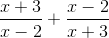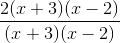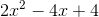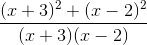Explanation:

To simplify the following, a common denominator must be achieved. In this case, the first term must be multiplied by (x+2) in both the numerator and denominator and likewise with the second term with (x-3).### Example Question #1 : Rational Expressions

Simplify the following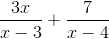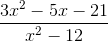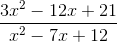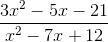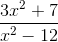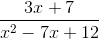Explanation:

Find the least common denominator between x-3 and x-4, which is (x-3)(x-4). Therefore, you have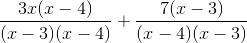.  Multiplying the terms out equals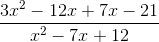. Combining like terms results in.

### Example Question #2 : Expressions

Simplify the following expression: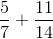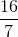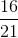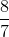Explanation:

In order to add fractions, we must first make sure they have the same denominator.

So, we multiply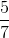by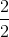and get the following: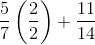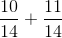Then, we add across the numerators and simplify: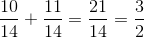### Example Question #1 : Expressions

Combine the following two expressions if possible.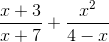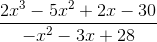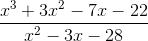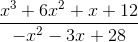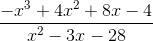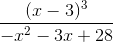Explanation:

For binomial expressions, it is often faster to simply FOIL them together to find a common trinomial than it is to look for individual least common denominators. Let's do that here: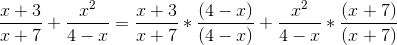FOIL and simplify.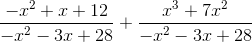Combine numerators.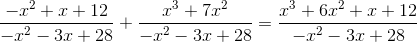Thus, our answer is### Example Question #4 : Expressions

Select the expression that is equivalent to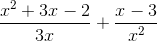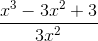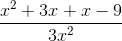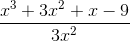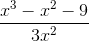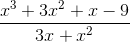Explanation:

To add the two fractions, a common denominator must be found. With one-term denominators, it is easier to simply find the least common denominator between them and multiply each side to obtain it.

In this case, the least common denominator betweenandis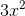. So the first fraction needs to be multiplied byand the second by: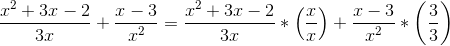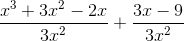Now, we can add straight across, remembering to combine terms where we can.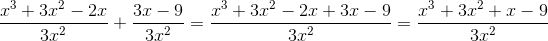So, our simplified answer is